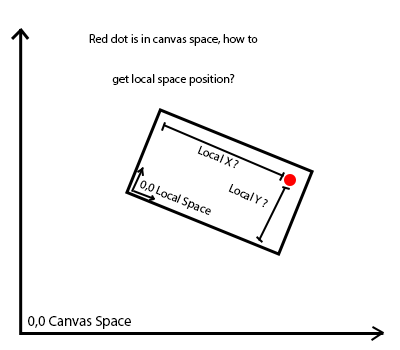# Convert Canvas Space point to local space of a rotated rect?

I got a quite tricky problem:

I have my mouse position and my rectangle position in canvas space(screen space but with adjusted scaling). Now I want my mouse position converted to local space of the rectangle. Problem is, the rectangle can be rotated. Without rotation its quite simple and works nicely.

Heres a graphic for better demonstration:Solved with help from stackoverflow. The solution is as follows:

``````    var mapRect = MapContentTransform.rect;

// Canvas Space Map Center
var mapPositionCenter = CanvasRectTransform.InverseTransformPoint(MapContentTransform.position);
mapPositionCenter.x += CanvasRectTransform.rect.width / 2.0f;
mapPositionCenter.y += CanvasRectTransform.rect.height / 2.0f;
// Mouse Position in Canvas Space
Vector2 mousePositionCanvasSpace = CalculateScreenspacePositionCanvasSpace(new Vector2(Input.mousePosition.x, Input.mousePosition.y));

// Rotation angle of the Map in Radians
float theta = -(MapContentTransform.eulerAngles.z - 360.0f) * Mathf.Deg2Rad;

var rotatedX = ((mousePositionCanvasSpace.x - mapPositionCenter.x) * Mathf.Cos(theta) -
(mousePositionCanvasSpace.y - mapPositionCenter.y) * Mathf.Sin(theta)) + mapRect.width / 2.0f;

var rotatedY = ((mousePositionCanvasSpace.y - mapPositionCenter.y) * Mathf.Cos(theta) +
(mousePositionCanvasSpace.x - mapPositionCenter.x) * Mathf.Sin(theta)) + mapRect.height / 2.0f;
``````

I had to adjust for Unity’s Counter-Clockwise UI rotation(The formula requires Clockwise!) as well as the offset from the center of the rotated image since I want the coordinates to be from 0 to image.size.

Hi @TriNityGER
Scaling nor rotation does not matter here I think. This is more like matter of space conversion, and you are interested in point’s location in Canvas RectTransform space, transformed into your other RectTransform’s space:

``````public RectTransform refItemRt;
public RectTransform placedItemRt;
public RectTransform targetSpaceRt;

void Update ()
{
var pos = targetSpaceRt.InverseTransformPoint(refItemRt.position);
placedItemRt.localPosition = pos;
}
``````